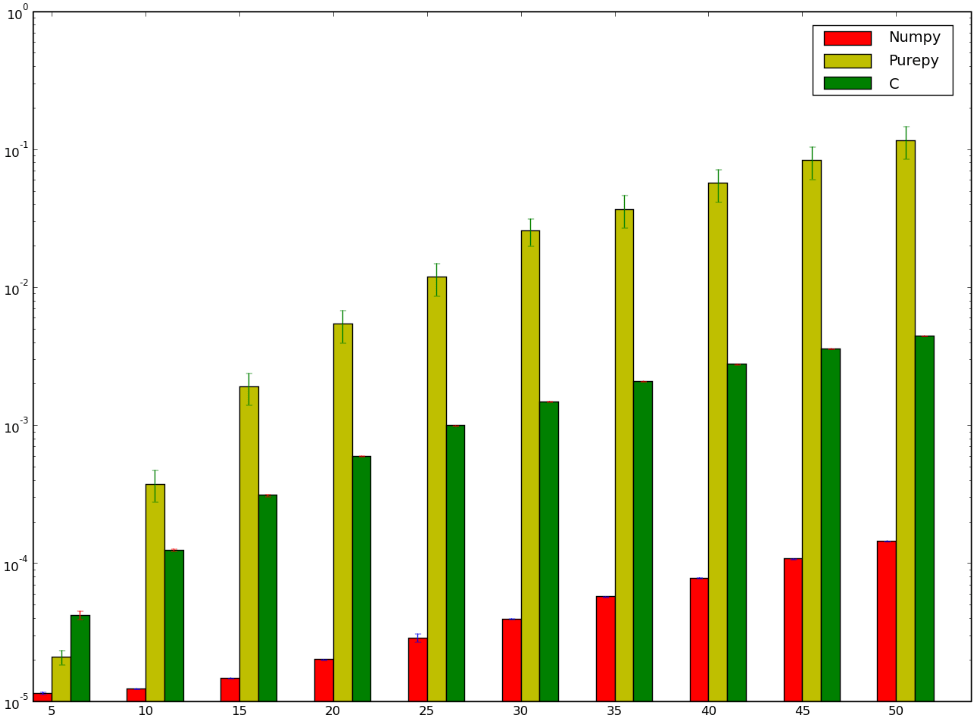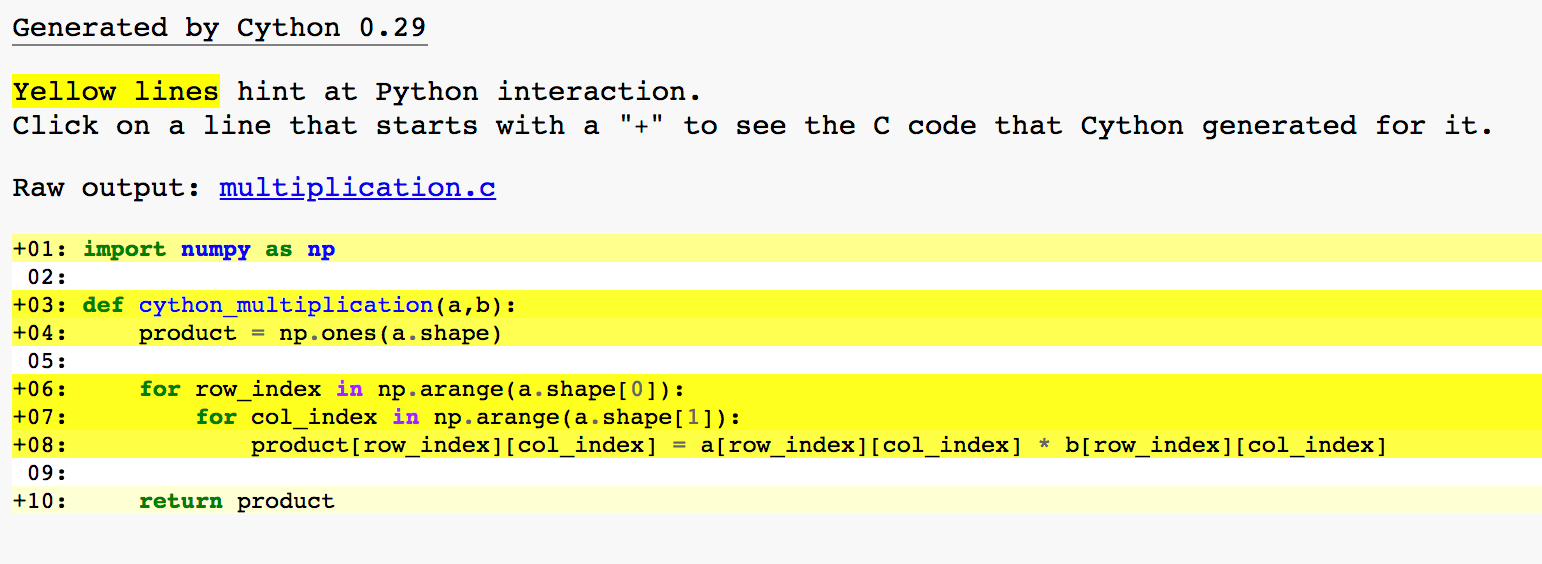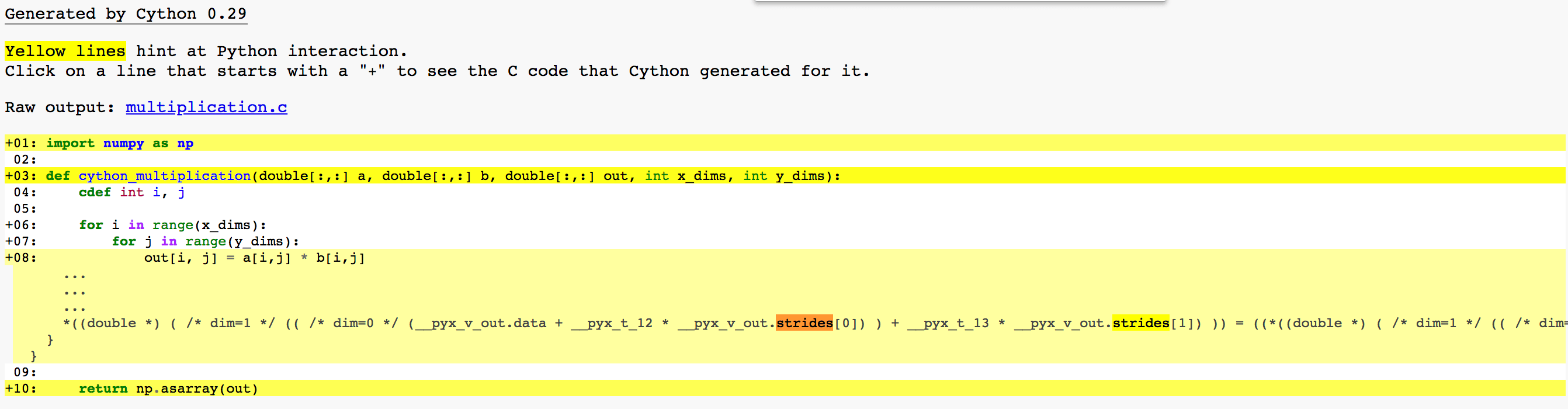# Code Mechanic: Numpy Vectorization

Reading Time: 15 minutesPython’s best known scientific computing libraries have lots of documentation about how to use them.

But how do the tools work under the hood? Google searches turn up fewer answers to this.

Luckily the tools are open source, so I can dive into the code myself.

I did a post about diving into the Scipy CSR Matrix. As it turns out, the code reconstitutes sparse arrays into dense ones with a pair of `for` loops in C++. It’s fast because C is fast.

But that got me thinking about vectorization in Numpy. Numpy arrays tout a performance (speed) feature called vectorization.

The generally held impression among the scientific computing community is that vectorization is fast because it replaces the loop (running each item one by one) with something else that runs the operation on several items in parallel. That technique is commonly called parallelization in the wider programming community.

But what if these lauded “vectorizations” are, at their core, just `for` loops in a fast language (namely C) without any parallel magic? Let’s find out.

### How do we know if Numpy’s speed is due to C or to parallelization?

Let’s do this the hard way, because the more gray hairs we give ourselves the better we’ll remember the lesson :).

We’ll write the loops in C and compare their speed to Numpy’s. If the loops are beating Numpy, then maybe Numpy is just loops. If Numpy beats the loops, maybe something more clever is going on in there.

Before we do this, let’s acknowledge that this has been done. Charles Menguy shared some results on StackOverflow in 2012:

I was trying to figure out the fastest way to do matrix multiplication and tried 3 different ways:

• Pure python implementation: no surprises here.
• Numpy implementation using `numpy.dot(a, b)`
• Interfacing with C using `ctypes` module in Python.

Charles’ test purported to demonstrate that numpy operates faster than a straight looping C implementation. We’re not going to just leave it at that, but I figured I’d share the chart because charts are pretty:### Wait, why can’t we just leave it at that?

The thing is, the implementation deployed for this particular test is more naive than a looping implementation has to be. Granted it is meant to work for an array of arbitrary size and dimension, but it doesn’t do that, so I cannot give it full marks for this. Additionally, the stride is implicitly, rather than explicitly, defined. Also, the `matmult` method does a multiplication operation that could benefit from a structural efficiency by transposing one of the factors, and this implementation doesn’t do that (you’ll see why this is useful in a diagram we’ll draw later).

I redid the C with an explicitly defined matrix size and dimensionality. Matrix-handling libraries commonly have separate array manipulation implementations not only for separate numbers of dimensions, but even for separate data types.

I also added a struct for object clarity.

### C Experiment Number 1: Straight C with ctypes

Here’s all the code on Github, if you’re into that kind of thing.

Here are the multiplication methods:

and the calls:

Results:It looks like our straight Python takes 4 seconds (ouch), followed by the Numpy implementation at 0.013 seconds, followed by our C at 0.009 seconds. These numbers differ a little each time I run this code, but these results are typical.

Worth noting: the C timing is faster than the Numpy timing right now, but as you can see, the C time does not include loading the data into a C array and then back into a python-usable array. Since I do that element by element with python, it wouldn’t be a fair comparison to the C implementation with that in there. But since Numpy takes and returns a python-usable collection, this timing method isn’t exactly fair to Numpy.

### C Experiment Number 2: Cython Conversion of Straight Python

Let’s try this with Cython instead of with ctypes. Cython handles its own python to C conversion, so we will literally copy our python implementation into a Cython rig first and see if we pick up any speed that way.

Python code converting to Cython:

updated execution:

Updated results:Yow. Our Cython version takes 3.2 seconds—better than the straight Python without conversion, but nowhere near as fast as the straight C or Numpy.

Of course, the C part is still faster. That’s why the Cython one can load a python collection into C, run the transformation, unload the C array back into python, and still finish faster than the python implementation did despite having an extra step at the beginning and the end.

Here’s the Python interaction heat map for this code. We have a lot of Python interaction going on here as indicated by the yellow highlights: a middle yellow line on the import statement, then five lines of intense yellow, and a faint yellow on that return value. In fact, not a single line of our code avoids Python interaction.The Python interaction is a pretty gaping speed leak. Let’s do this same thing in a more C-ish manner and see if it’s any faster. Note that we have even defined our API in C style: we pass a return value, and we pass the number of dimensions as integers. I got lazy at the end and used `np.asarray()` to convert my return value. Sue me.

### C Experiment Number 3: Cython, But Make it C-ish

New code for Cython:

Updated execution:

Updated results:We’re down to 0.015 seconds on that Cython code! We picked up a lot of speed dropping straight into C. Python interaction analysis is much less yellow:The remaining yellow is:

Line 1 (middle yellow): Import a Python API
Line 3:(intense yellow) Take in 5 Python objects
Line 8:(faint yellow) Convert 3 Python collections to C arrays
Line 10:(middle yellow) Convert a C object into a Python collection.

Now we have something a lot faster than pure Python that takes in the Python inputs and returns a Python-manipulable collection.

But numpy’s multiplication is still clocking in at a 33% time savings off this.

So, the emperor is wearing clothes! The speed of C alone doesn’t fully explain Numpy’s speed. Numpy did all that same stuff faster than a naive looping C implementation.

### What sort of implementation would mean less looping?

Well, instead of looping over elements in a blocking synchronous queue, it might take all the independent operations and do them at once.

For elementwise multiplication, for example, all of the calculations are independent. You don’t need the result of any particular element by element multiplication to calculate any of the others. One could, theoretically, open as many threads as there are elements in the base arrays and run all the calculations concurrently. The sky’s the limit! Well, not really: your CPU is the limit. But you get the idea.

The approach is even viable if some of the calculations do depend on one another. Take the dot product as an example: to calculate a dot product, one takes two arrays with corresponding numbers of elements in their inner dimensions, calculates an array with dimensions corresponding to the factors’ outer dimensions, then sums up those products to produce a scalar result.

In this case, the sum operation needs the multiplication operations to finish before it can finish because it needs to sum up the products. So we cannot put each element in its own thread and call it a day. We could, however, parallelize the multiplication operations, then run addition later.

Here’s a picture of a naive dot product implementation on the left, then an illustration of how we can imagine a parallelized version to work on the right. We don’t know at this point whether this is how vectorization works in Numpy, but this is how it could work if the code is indeed taking advantage of parallelization:A note: this is the thing I mentioned about transposing helping us out. When we transpose the second array for doing the dot product, we can now do the multiplication calculations element-wise by the outer dimension, rather than having to move over one dimension in the first factor and then loop over the other one in the other factor. We can even use the same indices this way.

So, the million dollar question: does Numpy do this? Let’s see what we can learn about how this works, first from a few papers and then from the code itself.

### The Scuttlebutt

If you’re looking to familiarize yourself with Numpy use cases, I can recommend this paper by Van der Walt, Colbert, and Varoquaux as a useful rundown. But it doesn’t address how the features work underneath the API.

If you want to know all the gritty implementation details, the piece you’re looking for is Beautiful Code, Chapter 19: “Multidimensional Iterators in Numpy” by Travis E. Oliphant.

I won’t quote the entire chapter in this post, but the chapter first discusses the historical challenges of iteration over memory addresses, then explains the pieces necessary for a general solution. With that explanation complete, we arrive at the following signature for the base iterator object in Numpy. I have added arrows with brief explanations, but for full context I recommend the chapter itself:

Sure enough, the `PyArrayIterObject`​ appears in the Numpy elementwise iteration function:

By the way folks, that right there is how you do attribution in code.

We also see the struct in use in the C-converted code from our own elementwise multiplication function. Here, we see the a PyArrayIterObject generated to manage iteration over our output array, `out`:Mind you, this is the base iterator for the Numpy arrays. It goes element by element in a given dimension, just like the iterators you’re used to. The design of this particular iterator takes advantage of a number of performance enhancements related to memory usage and compute time.

For example:

A common motif in NumPy is to gain speed by concentrating optimizations on the loop over a single dimension where simple striding can be assumed. Then an iteration strategy that iterates over all but the last dimension is used. This was the approach introduced by NumPy’s predecessor, Numeric, to implement the math functionality.

In NumPy, a slight modification to the NumPy iterator makes it possible to use this basic strategy in any code. The modified iterator is returned from the constructor as follows:

```   it = PyArray_IterAllButAxis(array, &dim).
```

The PyArray_IterAllButAxis function takes a NumPy array and the address of an integer representing the dimension to remove from the iteration. The integer is passed by reference (the & operator) because if the dimension is specified as –1, the function determines which dimension to remove from iteration and places the number of that dimension in the argument. When the input dimension is –1, the routine chooses the dimension with the smallest nonzero stride.

Another choice for the dimension to remove might have been the dimension with the largest number of elements. That choice would minimize the number of outer loop iterations and reserve the most elements for the presumably fast inner loop. The problem with that choice is that getting information in and out of memory is often the slowest part of an algorithm on general-purpose processors.

As a result, the choice made by NumPy is to make sure that the inner loop is proceeding with data that is as close together as possible. Such data is more likely to be accessed more quickly during the speed-critical inner loop.

OK, so each loop should run fast. But to implement true parallelization, Numpy would need to run multiple iterators at the same time. How does Numpy handle multiple iterations within an array or collection of arrays?

#### Multiple Iterations

Another common task in NumPy is to iterate over several arrays in concert. For example, the implementation of array addition requires iterating over both arrays using a connected iterator so that the output array is the sum of each element of the first array [sic]multiplied by* each element of the second array.

*This is exactly what the chapter says. This paragraph starts by exemplifying elementwise addition in arrays, but then explains that as taking the sum of elementwise multiplication. Those two operations are not the same thing. I suspect what happened here is that the example was originally elementwise multiplication (that is, exactly what we are doing), but an editor suggested elementwise addition instead because addition is a sort of universal base case for scalar operations, and most of this paragraph got changed, but the explanation of the operation did not. We will operate under the assumption that this whole thing is about elementwise addition and “multiplied by” should say “and” (for addition of two elements).

So anyway, what does the chapter say we can do about that?

This can be accomplished using a different iterator for each of the input elements [the factor arrays] and an iterator for the output array in the normal fashion.

Alternatively, NumPy provides a multi-iterator object that can simplify dealing with several iterators at once.

This is where the parallelization would happen: multiple iterators running at the same time on an array or combination of arrays. In this case, we have an iterator for each individual input—an input being one of the addend arrays, rather than the elements thereof.

Here’s the method that returns us that multi-iterator object in Numpy:

I’m not seeing any split-and-parallelize logic in what I’m reading from `iterators.c` in the Numpy library.

There’s no mention of the iterations running concurrently in the chapter, either. The word “simultaneous” does come up in the context of broadcasting. That said, I interpret the author(s) to be saying that multiple broadcasting operations can be run on the same collection of inputs—not that any iteration are happening at the same time. My interpretation could be wrong. Read the chapter! Draw your own conclusions. Write comments about them.

Admittedly, element-wise threading for multiplication could be a lot of threads. It could be up to as many threads as the factors have elements, since all operations are independent. How about dot products, though? That’s a more contained number of potential simultaneous operations: one for each inner dimension of the factors, then a final one to add up all the products. It’s less complicated because there is less pressure to trade off between number of threads and number of operations run in a given thread. So how does Numpy do it? Here’s the code:

Whelp, that’s a loop.

So at least in these two cases, Numpy vectorization does not appear to be using parallelization.

That doesn’t mean it’s implemented wrong. I did no research into whether parallelization would, in fact, be simpler or faster in this case than what’s already happening. And the degree to which data scientists talk up Numpy’s speed tells me that, as far as many practitioners are concerned, Numpy’s existing optimizations are puh-lenty fast enough.

However, they’re not fast for the reason that data scientists say they are. They’re not running independent operations concurrently.

They’re looping fast. They’re looping really fast. They employ some genius optimizations to loop really really fast.

But they’re looping.

### Conclusion

Numpy vectorization has a reputation in the industry for being fast by capitalizing on parallelization.

Indeed, Numpy is fast. Its iterators compile back to C, but their implementation is fast for reasons other than C itself. The base iterator does an excellent job of encapsulating the kernel of commonality between collection operations. As technologists, we can benefit from studying this code as an example of thoughtful software design.

Moreover, that iterator demonstrates some really great choices to get the loops in C to run as fast as possible.

But, as far as I can tell from reading the code and its analysis, it does not capitalize on parallelization.

So what’s the lesson here? The lesson is much like the lesson we learned from studying the history of API design. The lesson is that just because lots of people think something, doesn’t mean it’s true. And if we investigate what we’re learning rather than take it at face value, sometimes the underlying truth can be just as interesting.

### If you love getting into the technical weeds as much as I do, I also recommend:

This three part series on the history of API design

This similar weeds adventure with the Scipy CSR matrix

Gettin’ reeeeeal pedantic about git commit messages

This site uses Akismet to reduce spam. Learn how your comment data is processed.